## Thursday, 7 August 2014

### chapter 8 - Worked Out Examples 1

 Example: 1
 (a) A bag contains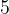$5$ red and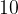$10$ white balls, all identical in shape and size. A person puts his hand in and without looking, pulls out a ball. What is the probability of it being red? (b) In the same bag, he puts his hand in and draws out a pair of balls. What is the probability of both of them being red?
 Solution: 1-(a)
It should be obvious that drawing a white ball is more likely than drawing a red one, since there are more white balls. Precisely,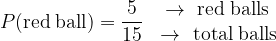$P({\rm{red}}\;{\rm{ball}}) = \dfrac{5}{{15}}\,\,\begin{array}{*{20}{c}} { \to \,\,{\rm{red}}\;{\rm{balls}}}\\ {\, \to \,\,{\rm{total}}\;{\rm{balls}}} \end{array}\;$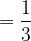$= \dfrac{1}{3}$ and$P({\rm{white}}\;{\rm{ball}}) = \dfrac{{10}}{{15}}\begin{array}{*{20}{c}} { \to {\rm{white balls}}}\\ { \to {\rm{total}}\;{\rm{balls}}} \end{array}\;$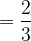$= \dfrac{2}{3}$
so that,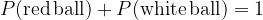$P({\rm{red\,ball}}) + P({\rm{white\,ball}}) = 1$ as expected
 Solution: 1-(b)
There are$5$ red balls, so there are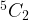${}^5{C_2}$ pairs of red balls possible. (Some readers might raise an objection here: if the balls are identical, how can there be${}^5{C_2}$ ways of forming pairs? There should be just one way, as discussed in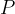$P$&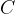$C$. These readers should try to appreciate the difference between number of pairs and number of ways of forming pairs. The former is${}^5{C_2}$, the latter is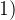$1)$.
The total number of pairs possible is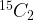${}^{15}{C_2}$. Thus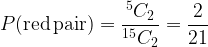$P({\rm{red\,pair}}) = \dfrac{{^5{C_2}}}{{^{15}{C_2}}} = \dfrac{2}{{21}}$
Similarly, we will have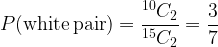$P ({\rm{white\,pair}}) = \dfrac{{^{10}{C_2}}}{{^{15}{C_2}}} = \dfrac{3}{7}$ and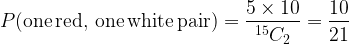$P ({\rm{one \,red,\, one \, white\, pair}})= \dfrac{{5 \times 10}}{{^{15}{C_2}}} = \dfrac{{10}}{{21}}$
Note that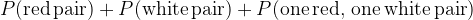$P ({\rm{red \, pair}}) + P ({\rm{white \, pair}}) + P ({\rm{one\, red,\, one\, white\, pair}})$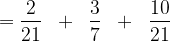$= \dfrac{2}{{21}}\;\; + \;\;\dfrac{3}{7}\;\; + \;\;\dfrac{{10}}{{21}}$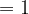$=1$
 Example: 2
There are two bags, one containing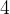$4$ white and$5$ black balls, and the other containing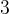$3$ white and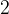$2$ black balls. A person draws one ball at random from each bag. Find the probability that
 (a) both balls are white (b) one ball is white and one is black
 Solution: 2-(a)
Let the two bags be labelled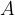$A$ and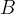$B$ respectively. Note that drawing a ball from$A$ is independent of drawing a ball from$B$. Thus,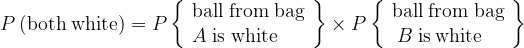$P\,({\rm{both}}\;{\rm{white}}) = P\left\{ \begin{array}{l} {\rm{ball}}\;{\rm{from}}\;{\rm{bag}}\\ A\;{\rm{is}}\;{\rm{white}} \end{array} \right\} \times P\left\{ \begin{array}{l} {\rm{ball}}\;{\rm{from}}\;{\rm{bag}}\\ \;B\;{\rm{is \,white }} \end{array} \right\}$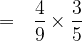$= \,\,\,\,\dfrac{4}{9} \times \dfrac{3}{5}$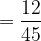$= \dfrac{{12}}{{45}}$
 Solution: 2-(b)
We note that the case {one white and one black} is possible in two ways: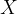$X$ : Ball from bag$A$ white, ball from bag$B$ black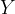$Y$ : Ball from bag$A$ black, ball from bag$B$ white
Note that$X$ and$Y$ are mutually exclusive events.
Thus,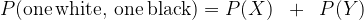$P ({\rm{one\, white,\, one \,black}}) = P(X)\;\; + \;\;P(Y)$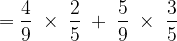$= \dfrac{4}{9}\; \times \;\dfrac{2}{5}\; + \;\dfrac{5}{9}\; \times \;\dfrac{3}{5}$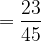$= \dfrac{{23}}{{45}}$
We could also have calculated this probability by noting that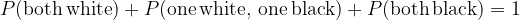$P ({\rm{both \, white}}) + P ({\rm{one \, white, \, one \, black}}) + P ({\rm{both \, black}}) = 1$
and since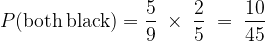$P({\rm{both\, black}}) = \dfrac{5}{9}\; \times \;\dfrac{2}{5}\; = \;\dfrac{{10}}{{45}}$, we have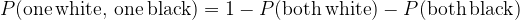$P({\rm{one\, white,\, one\, black}}) =1 - P ({\rm{both\, white}}) - P({\rm{both\, black}})$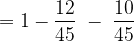$= 1 - \dfrac{{12}}{{45}}\; - \;\dfrac{{10}}{{45}}$$= \dfrac{{23}}{{45}}$